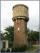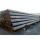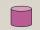Rectangle pool

Determine dimensions of open pool with a square bottom with a capacity 32 m3 to have painted/bricked walls with least amount of material.

Result

a =  4 m
c =  2 m

Solution:Leave us a comment of example and its solution (i.e. if it is still somewhat unclear...):Be the first to comment!To solve this example are needed these knowledge from mathematics:

Looking for help with calculating roots of a quadratic equation? Do you have a linear equation or system of equations and looking for its solution? Or do you have quadratic equation? Tip: Our volume units converter will help you with converion of volume units.

Next similar examples:

1. Swimming poolThe pool shape of cuboid is 299 m3 full of water. Determine the dimensions of its bottom if water depth is 282 cm and one bottom dimension is 4.7 m greater than the second.
2. Water reservoirThe cuboid reservoir contains 1900 hectoliters of water and the water height is 2.5 m. Determine the dimensions of the bottom where one dimension is 3.2 m longer than the second one.
3. EquationEquation ? has one root x1 = 8. Determine the coefficient b and the second root x2.
4. RootsDetermine the quadratic equation absolute coefficient q, that the equation has a real double root and the root x calculate: ?
5. DiscriminantDetermine the discriminant of the equation: ?
6. Square root 2If the square root of 3m2 +22 and -x = 0, and x=7, what is m?Quadratic equation ? has roots x1 = 80 and x2 = 78. Calculate the coefficients b and c.
8. TubesIron tubes in the warehouse are stored in layers so that each tube top layer fit into the gaps of the lower layer. How many layers are needed to deposit 100 tubes if top layer has 9 tubes? How many tubes are in bottom layer of tubes?Find the roots of the quadratic equation: 3x2-4x + (-4) = 0.
10. Theorem proveWe want to prove the sentence: If the natural number n is divisible by six, then n is divisible by three. From what assumption we started?
11. Solve 3Solve quadratic equation: (6n+1) (4n-1) = 3n2
12. Cuboid edges in ratioCuboid edges lengths are in ratio 2:4:6. Calculate their lengths if you know that the cuboid volume is 24576 cm3.
13. Evaluation of expressionsIf a2-3a+1=0, find (i)a2+1/a2 (ii) a3+1/a3
14. Cylinder diameterThe surface of the cylinder is 149 cm2. The cylinder height is 6 cm. What is the diameter of this cylinder?
15. Reciprocal equation 2Solve this equation: x + 5/x - 6 = 4/11
16. Cylinder - areaThe diameter of the cylinder is one-third the length of the height of the cylinder. Calculate the surface of cylinder if its volume is 2 m3.If 5x + x² > 100, then x is not